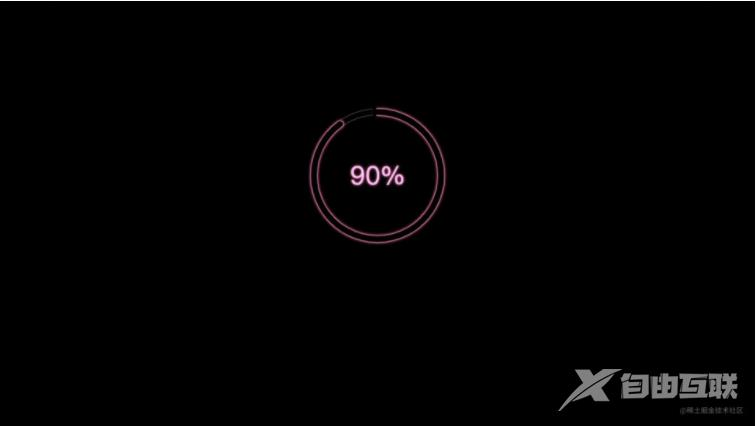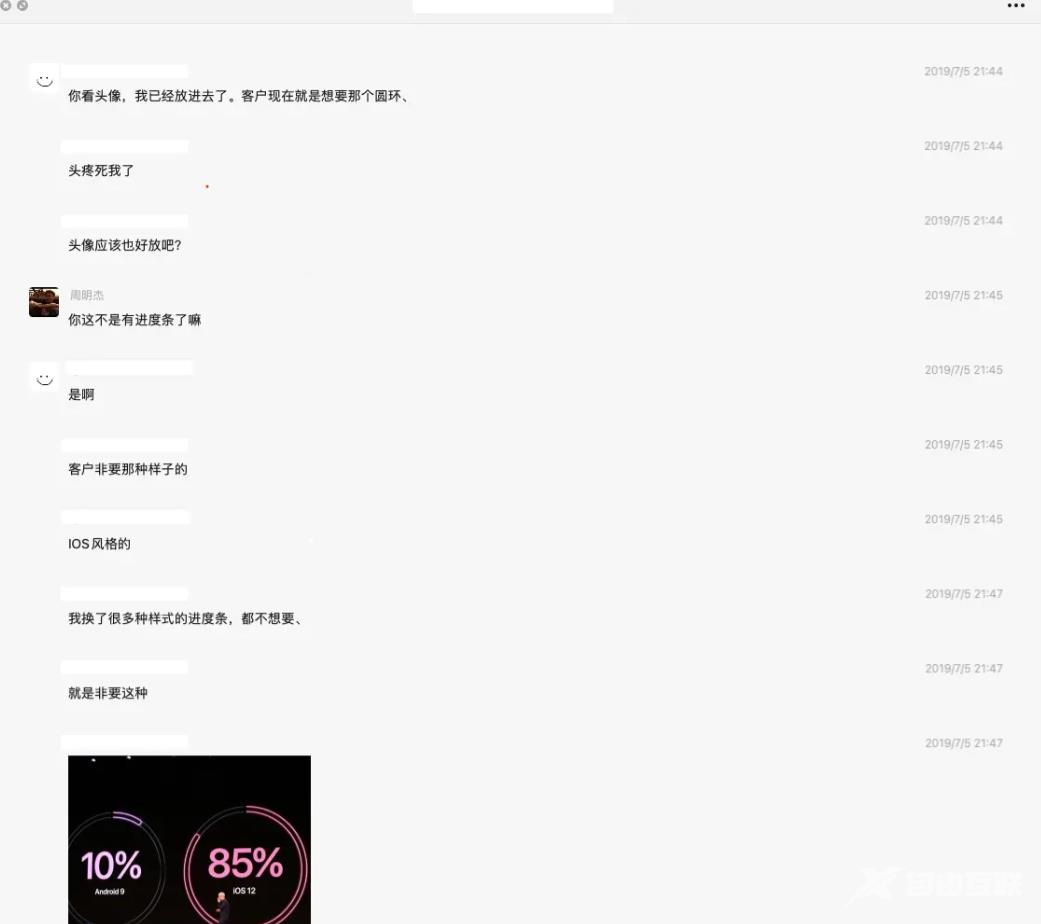﻿ JavaScriptcanvas复刻苹果发布会环形进度条 - 自由资讯

# JavaScriptcanvas复刻苹果发布会环形进度条

• 前言
• 基础 Dom 结构
• 基本变量
• 画圆
• 画弧线
• 让画面动起来
• 总结

## 前言

canvas 真是一个好东西，它给前端插上了想象的翅膀，伴随着 h5 而来，将 web 代入了新的领域，基于canvas 技术实现的各种酷炫效果和2d、3d 游戏，也让浏览器能承载更加强大的功能。尤其是它性能还很好，搞游戏再合适不过了，我就喜欢用 canvas 写一些小游戏玩。## 基础 Dom 结构

```<div class="container">
<canvas id="canvas" width="600" height="600">
<p>抱歉，您的浏览器不支持canvas</p>
</canvas>
</div>```

## 基本变量

```let canvas = document.getElementById("canvas")
let ctx = canvas.getContext("2d") // 上下文对象
let circleX = canvas.width / 2 // 中心x坐标
let circleY = canvas.height / 2 // 中心y坐标
let radius = 100 // 圆环半径
let percent = 90 // 最终百分比
let lineWidth = 1 // 圆形线条的宽度
let fontSize = 42 // 字体大小
```

## 画圆

```// 画圆
function circle(cx, cy, r) {
ctx.beginPath()
// ctx.moveTo(cx, cy-r-10)
ctx.lineWidth = lineWidth
ctx.strokeStyle = "#666"
ctx.lineCap = "round"
ctx.arc(cx, cy, r + 10, 0, (Math.PI / 180) * 360)
ctx.moveTo(cx + r, cy)
// ctx.moveTo(cx, cy-r)
ctx.arc(cx, cy, r, 0, (Math.PI / 180) * 360)
ctx.stroke()
}```

## 画弧线

```// 画弧线
function sector(cx, cy, r, startAngle, endAngle, anti) {
ctx.beginPath()
ctx.moveTo(cx, cy - r - 5) // 从圆形底部开始画
ctx.lineWidth = 12
ctx.strokeStyle = "#ffccff"
// ctx.fillStyle = '#ffccff'
// 圆弧两端的样式
ctx.lineCap = "round"

// 圆弧
ctx.arc(
cx,
cy,
r + 5,
startAngle * (Math.PI / 180.0) - Math.PI / 2,
endAngle * (Math.PI / 180.0) - Math.PI / 2,
anti
);
ctx.moveTo(cx, cy - r) // 从圆形底部开始画
ctx.moveTo(cx, cy - r) // 从圆形底部开始画
ctx.stroke()
}```

## 让画面动起来

```// 刷新
// 清除canvas内容
ctx.clearRect(0, 0, circleX * 2, circleY * 2)

// 中间的字
ctx.font = fontSize + "px April"
ctx.textAlign = "center"
ctx.textBaseline = "middle"
ctx.fillStyle = "#ffccff"
ctx.fillText(parseFloat(n).toFixed(0) + "%", circleX, circleY)

// 圆形

// 圆弧
sector(circleX, circleY, radius, 0, (n / 100) * 360)
sector2(circleX, circleY, radius, 0, (n / 100) * 360)
// 遮盖
}

// 更新进度
function changeProcess(val, times) {
return new Promise(function (resolve, reject) {
setTimeout(function () {
resolve()
}, times)
})
}

// 循环
async function loop(val) {
while (true) {
for (let i = 0; i < val; i++) {
await changeProcess(i + 1, 1000);
}
return
}
}

loop(percent)
```

﻿